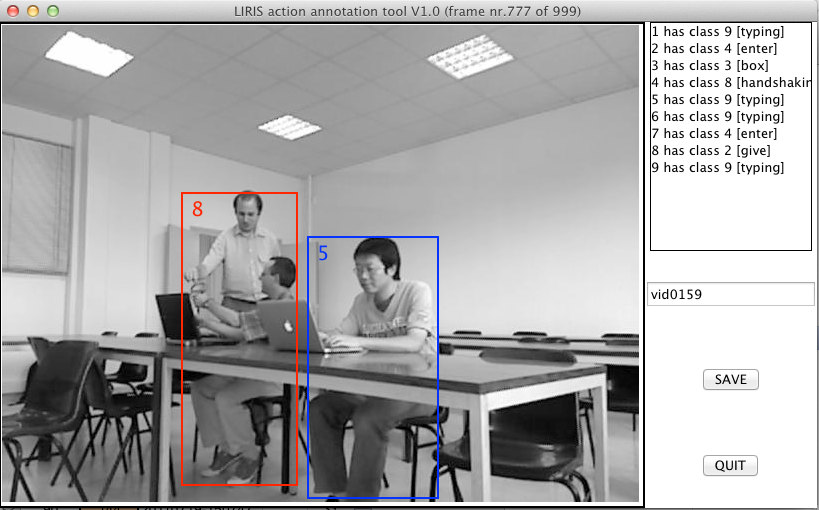# The LIRIS human activities dataset - XML file format

Main page | download | database structure | annotations in XML | camera calibration | annotation tools | evaluation tools

You need to cite the following journal paper in all publications including results for which you used the LIRIS dataset:.

C Wolf, J. Mille, E. Lombardi, O. Celiktutan, M. Jiu, E. Dogan, G. Eren, M. Baccouche, E. Dellandrea, C.-E. Bichot, C. Garcia, B. Sankur, Evaluation of video activity localizations integrating quality and quantity measurements, To appear in Computer Vision and Image Understanding, 2014.

## Annotation and result XML file format

The XML file format describing ground truth as well results is simply and self explaining, see the example below. A single video is described per XML file. The results are organized into actions, each of which is assigned a running number starting with 1 as well as an action class number. The results themselves are lists of bounding boxes, which are continguous in time (no holes).```<?xml version="1.0" encoding="UTF-8"?/>
<tagset/>
<video/>
<videoName/>dataset/vid0001</videoName/>
<action nr="1" class="3"/>
<bbox x="40" y="30" width="50" height="101" framenr="34"//>
<bbox x="41" y="31" width="51" height="105" framenr="35"//>
<bbox x="41" y="29" width="52" height="101" framenr="36"//>
<bbox x="41" y="30" width="51" height="104" framenr="37"//>
<bbox x="42" y="31" width="49" height="102" framenr="38"//>
<bbox x="42" y="33" width="51" height="103" framenr="39"//>
<bbox x="42" y="32" width="51" height="100" framenr="40"//>
<bbox x="42" y="33" width="52" height="100" framenr="41"//>
<bbox x="41" y="29" width="51" height="101" framenr="42"//>
<bbox x="41" y="31" width="51" height="101" framenr="43"//>
<bbox x="41" y="30" width="52" height="100" framenr="44"//>
</action/>
<action nr="2" class="9"/>
<bbox x="212" y="43" width="120" height="87" framenr="342"//>
<bbox x="211" y="42" width="121" height="86" framenr="343"//>
<bbox x="209" y="43" width="119" height="86" framenr="344"//>
<bbox x="208" y="42" width="123" height="86" framenr="345"//>
<bbox x="209" y="44" width="124" height="87" framenr="346"//>
<bbox x="207" y="42" width="123" height="87" framenr="347"//>
<bbox x="210" y="42" width="123" height="87" framenr="348"//>
<bbox x="212" y="43" width="124" height="86" framenr="349"//>
<bbox x="211" y="43" width="119" height="88" framenr="350"//>
</action/>
</video/>
</tagset/>
```

## Organization, contact

The dataset was collected and produced by members of the LIRIS Laboratory, CNRS, France
Christian Wolf, Julien Mille, Eric Lombardi, Bülent Sankur (BUSIM, Bogazici University, Turkey), Emmanuel Dellandréa, Christophe Garcia, Charles-Edmond Bichot, Mingyuan Jiu, Oya Celiktutan, Moez Baccouche

Send questions to christian.wolf (at) liris.cnrs.fr

You need to cite the following journal paper in all publications including results for which you used the LIRIS dataset:.

C Wolf, J. Mille, E. Lombardi, O. Celiktutan, M. Jiu, E. Dogan, G. Eren, M. Baccouche, E. Dellandrea, C.-E. Bichot, C. Garcia, B. Sankur, Evaluation of video activity localizations integrating quality and quantity measurements, In Computer Vision and Image Understanding (127):14-30, 2014.